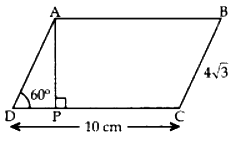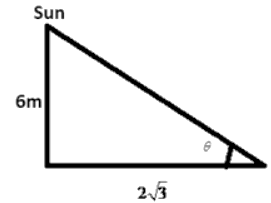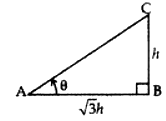Class 10 Maths Multiple Choice Questions of Application of Trigonometry with Answers. MCQ Questions for class 10 Maths with Answers was Prepared for students based on new patterns of exams. Applications of Trigonometry MCQ questions will assist you in gaining good marks within the examinations and also cracking competitive exams.

“Some applications of trigonometry” from the textbook deals with how trigonometry helps to find the height and distance of different objects without any measurement.

Learn below the given MCQ Questions for revision and Clear your concept: –

1. The ____ of an object is the angle formed by the line of sight with the horizontal when the object is below the horizontal level.

(a) line of sight
(b) angle of elevation
(c) angle of depression
(d) none of these

2. The angle of elevation of the sun, when the length of the shadow of a tree is equal to the height of the tree, is:

(a) 45°
(b) 60°
(c) 30°
(d) None of these

3. If the height of a tower and the distance of the point of observation from its foot, both, are increased by 10%, then the angle of elevation of its top

(a) increases (b) decreases
(c) remains unchanged
(d) have no relation.

4. …. is used for finding the heights and distances of various objects, without actually measuring them.

(a) Statistics
(b) Trigonometry
(c) Geometry
(d) None of the above

5. The line drawn from the eye of an observer to the point in the object viewed by the observer is ———

(a) Line of sight
(b) Line segment
(c) Line of contact
(d) None of the above

6. The height or length of an object, or the distance between two distant objects can be determined with the help of ————

(a) Trigonometric identities
(b) Trigonometric ratios
(c) Trigonometric formulae
(d) None of the above

7. A girl sitting on the balcony is looking down at a flower pot placed on a stair of the temple. The angle so formed by the line of sight with the horizontal is called the ———

(a) Angle of elevation
(b) Angle of depression
(c) Angle of repose
(d) None of the above

8. The angle of elevation of the top of a 15m high tower at a point 15m away from the base of the tower is ____ .

(a) 45°
(b) 30°
(c) 60°
(d) 90°

9. When we raise our head to look at the object, then the angle formed with the horizontal is ———

(a) Angle of elevation
(b) Angle of depression
(c) Angle of repose
(d) None of these

10. The angle of elevation of the top of a building from a point on the ground, which is 30 m away from the foot of the building, is 30°. The height of the building is:

(a) 10 m
(b) 30/√3 m
(c) √3/10 m
(d) 30 m

11. If the height of the building and distance from the building foot’s to a point is increased by 20%, then the angle of elevation on the top of the building:

(a) Increases
(b) Decreases
(c) Do not change
(d) None of the above

12. If a tower 6m high casts a shadow of 2√3 m long on the ground, then the sun’s elevation is:

(a)60°
(b)45°
(c)30°
(d)90°

13. The angle of elevation of the top of a building 30 m high from the foot of another building in the same plane is 60°, and also the angle of elevation of the top of the second tower from the foot of the first tower is 30°, then the distance between the two buildings is:

(a) 10√3 m
(b) 15√3 m
(c) 12√3 m
(d) 36 m

14. The angle formed by the line of sight with the horizontal when the point being viewed is above the horizontal level is called:

(a)Angle of elevation
(b)Angle of depression
(c)No such angle is formed
(d)None of the above

15. From a point on the ground, which is 15 m away from the foot of the tower, the angle of elevation of the top of the tower is found to be 60°. The height of the tower standing straight is:

(a)15√3
(b)10√3
(c)12√3
(d)20√3

16. At some time of the day, the length of the shadow of a tower is equal to its height. Then the angle of elevation of the sun is

(a) 45°
(b) 60°
(c) 120°
(d) 30°

17. A tower stands vertically on the ground. From a point on the ground which is 20 m away from the foot of the tower, the angle of elevation of its top is found to be 60°. Then the height of the tower is

(a) 20$$\sqrt3$$
(b) 30$$\sqrt3$$
(c) 20
(d) 40$$\sqrt3$$

18. When the length of shadow of a vertical pole is equal to √3 times of its height, the angle of elevation of the Sun’s altitude is

(a) 30°
(b) 45°
(c) 60°
(d) 15°

19. In given figure, ABCD is a || gm. The lenght of AP is(a) 2 cm
(b) 4 cm
(c) 6 cm
(d) 8 cm

20. In figure given ABCD is a rectangle, the value of CE is(a) 1 cm
(b) 2 cm
(c) 3 cm
(d) 4 cm

1. Answer: (c) angle of depression

Explanation: Since

tan θ = h/x

Where h is height and x is distance from tower,

If both are increased by 10%, then the angle will remain unchanged.

5. Answer: (a) Line of sight

7. Answer: (b) Angle of depression

Explanation: ∵ Height of tower = distance of point from the base

∴ Angle of elevation = 45°.

9. Answer: (a) Angle of elevation

Explanation: Say x is the height of the building.

a is a point 30 m away from the foot of the building.

Here, height is the perpendicular and distance between point a and foot of building is the base.

The angle of elevation formed is 30.

Hence, tan 30 = perpendicular/base = x/30

1/√3 = x/30

x=30/√3

11. Answer: (c) Do not change

Explanation: We know, for an angle of elevation θ,

Tan θ = Height of building/Distance from the point

If we increase both the value of the angle of elevation remains unchanged.

Explanation: As per the given question:Hence,

tan θ = 6/2√3

tan θ = √3

tan θ = tan60°

θ = 60°

Explanation: As per the given question:Hence,

tan60° = 30/x

√3 = 30/x

x = 30/√3

x = 10√3m

Explanation: We know:

Tan (angle of elevation) = height of tower/its distance from the point

Tan 60 = h/15

√3 = h/15

h=15√3

Explanation: Let the length of shadow =x

⇒ Height =x

Angle =A

so, tanA= $$\mathrm{\frac{x}{x}}=1$$

tan A = tan 45°

So, A= 45°

17. Answer: (a) (a) 20$$\sqrt3$$

Explanation: Let AB be the tower standing vertically on the ground and O be the position of the obsrever we now have:
OA = 20m, ∠OAB = 90° and ∠AOB = 60°
Let
AB = hmNow, in the right ΔOAB,we have:
$$\frac{AB}{OA}-tan60^o=\sqrt3$$
$$\Rightarrow \frac{h}{20}= \sqrt3$$
$$\Rightarrow h=20\sqrt3$$

Explanation: Let the height of the vertical pole, BC = h m
∴ Shadow AB = √3 h m and the angle of elevation ZBAC = θIn rt$$\Delta ABC,\,tan\theta=\frac{BC}{AB}=\frac{h}{\sqrt3h}=\frac{1}{\sqrt3}=tan30^o$$
∴ θ = 30°
Hence the Sun’s altitude is 30°

Explanation: Since ABCD is a || gm
∴ AD = BC = 4√3
In rt ∆APD, sin 60° = $$\frac{AP}{AD}$$
⇒ √3/2=AP/4√3
⇒ 2AP = 4 × 3 = 12
∴ AP = 6 cm

Explanation: Since ABCD is a rectangle
∴ BC = AD = 8 cm and B = 90°
In rt ∆CBE, cos 60° = $$\frac{CE}{BC}$$
⇒ $$\frac{1}{2} = \frac{CE}{8}$$
∴ CE =$$\frac{ 8}{2}$$ = 4 cm